# AP Calculus BC Practice Test 1

### Test Information10 questions20 minutes

Calculator Disallowed

1. If 7 = xy - exy, then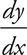=

2. The volume of the solid that results when the area between the curve y = ex and the line y = 0, from x = 1 to x = 2, is revolved around the x-axis is

3.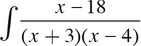dx =

4. If y = 5x2 + 4x and x = ln t, then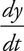=

5. If f(x) =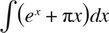6. The tangent line to the curve y = x3 - 4x + 8 at the point (2, 8) has an x-intercept at

7. The graph in the xy-plane represented by x = 3sin(t) and y = 2cos(t) is

8.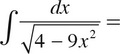9.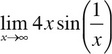is

10. The position of a particle moving along the x-axis at time t is given by x(t) = ecos(2t), 0 ≤ tπ. For which of the following values of t will x′(t) = 0?

I. t = 0

II. t =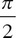III. t = π